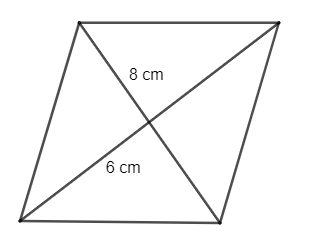Courses
Courses for Kids
Free study material
Free LIVE classes
MoreLIVE
Join Vedantu’s FREE Mastercalss

# Find the area of rhombus whose diagonals are of length 8cm and 6cm--A.20 $c{{m}^{2}}$B.42 $c{{m}^{2}}$C.14 $c{{m}^{2}}$D.24 $c{{m}^{2}}$Verified
334.8k+ views
Hint: we know that the formula for area of rhombus is given by $=\dfrac{1}{2}\times {{d}_{1}}\times {{d}_{2}}$if the diagonals are of length ${{d}_{1}}$and ${{d}_{2}}$. We know the diagonals of rhombus so by that we can get the area of rhombus.

The area of a rhombus can be defined as the amount of space enclosed by a rhombus in a two-dimensional space. To recall, a rhombus is a type of quadrilateral projected on a two dimensional (2D) plane, having four sides that are equal in length and are congruent. It is also known as equilateral quadrilateral because all its four sides are equal in nature.

 Formulas to Calculate Area of Rhombus Using Diagonals A = ½ × d1 × d2 Using Base and Height A = b × h Using Trigonometry A = b2 × Sin(a)

Given the diagonals of a rhombus are of length 8cm and 6cm.We know that the area of rhombus is given by $\dfrac{1}{2}\times {{d}_{1}}\times {{d}_{2}}$if diagonals are of length ${{d}_{1}}$and ${{d}_{2}}$
$=\dfrac{1}{2}\times {{d}_{1}}\times {{d}_{2}}$. . . . . . . . . . . . . . . . . . . . . . . . . . . . . . . . . . . . . . . . . (1)
$=\dfrac{1}{2}\times 8\times 6c{{m}^{2}}$. . . . . . . . . . . . . . . . . . . . . . . . . . . . . . . . . . . . . . . (2)
$=24c{{m}^{2}}$. . . . . . . . . . . . . . . . . . . . . . . . . . . . . . (3)
Hence the area of given rhombus is $24c{{m}^{2}}$

So, the correct answer is “Option A”.

Note: The rhombus is a flat shape with 4 straight sides that are of equal length. In rhombus all opposite sides are parallel and opposite angles are equal. All rhombus are parallelograms, but not all parallelograms are rhombuses. All squares are rhombuses, but not all rhombuses are squares. Diagonals of a rhombus bisect each other at right angles.
Last updated date: 22nd Sep 2023
Total views: 334.8k
Views today: 3.34k